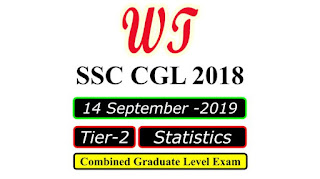Type Here to Get Search Results !

# Download SSC CGL 2018 Tier 2, Statistics Paper, 14 September 2019

Download SSC (Staff Selection Commission) CGL (Combined Graduate Level Examination) 2018 Tier 2, Statistics Paper Was Held on 14 September 2019.SSC CGL 2018 Tier 2 Statistics 14 Sep 2019 Paper PDF

Q1:- The prices (in Rs.) of different yarns (Per Kg) in two consecutive years are as follows.
Commodity        Silk    Cotton    Jute    Rayon
Price (in 2016)    600    700        400     300
Price (in 2017)    700    600        480     270
By simple aggregative method, the net price changes in % is:
a.    Net increase of 2.5% in price
b.    Net increase of 2 % in price
c.    Net decrease of 2% in price
d.    Net decrease of 2.5% in price

Q2:- The avenge working hours per month of the staff aged over 50 yens in a factory were 160 and that of the staff aged under 50 years were 210. The mean working hour per month of all the staff was 200. The ratio of the number of the staff aged over 50 to that of the staff aged under 50 is:
a.    3 : 1
b.    2 : 1
c.    1 : 3
d.    1 : 4

Q3:- The 4th decile for the given data is:
X    f
0    1
1    9
2    26
3    59
4    72
5    52
6    29
7    7
8    1
a.    5
b.    3
c.    4
d.    7

Q4:- The mean deviation about median for the given data.
52,56,66,70,75,80,82 is:
a.    9
b.    7
c.    3
d.    6

Q6:- The memory-less property is followed by which of the following continuous distribution:
a.    Continuous uniform distribution
b.    Normal distribution
c.    Gamma distribution
d.    Exponential distribution

Q12:- Which of the following methods is NOT used in computation of a seasonal index for time series?
a.    Method of averages
b.    Link relative method
c.    Moving average method If
d.    Mathematical equations

Q14:- For the study purpose. the mean of the observations is 148 gm and standard deviation is 17.4 gm. Approximately, the coefficient of variation equals to:
a.    11
b.    14
c.    12
d.    13

Q16:- Statistics is not applicable to _____ observation.
a.    Classified
b.    Group
c.    Individual
d.    Monotonic

Q17:- The mode (correct to two decimal place) for the given data is:
Class-interval    Frequency
0-10                      6
10-20                    9
20-30                    8
30-40                    14
40-50                    28
50-60                    20
60-70                    11
70-80                     9
a.    39.34
b.    46.36
c.    28
d.    52.54

SSC CGL 2018 Tier 2 All Papers